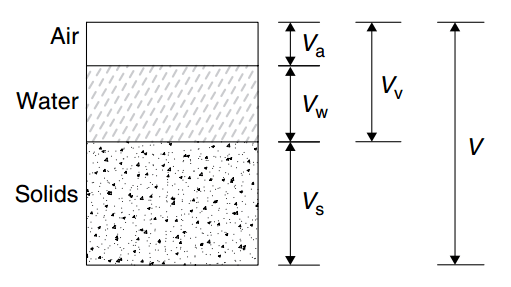# [土与基础] 土的物理性质指标 (Physical Properties of Soil)（1）土的质量密度 ρ Mass density

ρ = m/V

（2）土的重力密度 γ Weight density

γ = mg/V

（3）土的含水量 ω Moisture content

ω = mw/ms*100%

（4）土的相对密度（比重） ds Relative  density）（Specific Gravity, Gs

ds =ms/( Vs ρ) = ρs

Gs = Ws / Ww= ds

（5）土的干密度 ρd Dry  density

ρd=ms/V

（6）土的饱和密度 ρsat Saturated density

ρsat = ( ms + Vvw )/ V

（7）土的有效重度 γ′Submerged density

γ′ = ( ms + Vvw )/ V – V*γw /V = γ sat – γw

（8）土的孔隙比 e（Voids Ratio

e = Vv / V

e = dw*ρw (1 + w ) / ρ – 1

（9）土的孔隙率 n（Porosity, n

n = Vv / V *100%

n = e / (1+e) *100%

（10）土的饱和度 Sr（Degree of Saturation, Sr

Sr = Vw / Vv

• 相关博文 ( Related Topics)